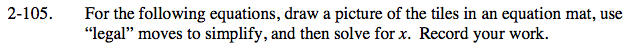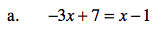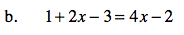### Home > CAAC > Chapter 2 > Lesson 2.2.2 > Problem2-105

2-105.
1. For the following equations, draw a picture of the tiles in an equation mat, use “legal” moves to simplify, and then solve for x. Record your work. Homework Help ✎

1. −3x + 7 = x − 1

2. 1 + 2x − 3 = 4x − 2Use tiles to illustrate the problem.

One strategy is to flip all of the tiles in the subtraction region into the addition region.

−3x + 7 = x + (−1)

Simplify by getting all of the variables on one side and all of the 1 and −1 tiles on the other side, eliminating zero pairs.

x = 2Use the same strategy as in part (a).

Use the eTool below to place the tiles on the blank expression mat to model the problem.
Click the link at the right to view full eTool version: 2-105 HW eTool.